Basics of Geometry
edHelper subscribers - Create a new printable

Number of Keys
 Select the number of different printables: 1 key 2 keys 3 keys 4 keys 5 keys

Number of Pages (for each key)
 Select the number of pages: 1 page 2 pages 3 pages 4 pages

Include an answer key (answer keys will be at the end of the printable)
High School Geometry
High School Geometry
Math

 Name _____________________________Date ___________________ (Key 1 - Answer ID # 0814324)
1.

Points M, N, and X are collinear and X is between M and N. If MN = 28 and the ratio between MX and NX is 3:4, find the length of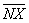.

2.

Find the distance between the two points. (a) G(6, 2), H(6, -4) (b) M(5, -7), N(-3, 4) (c) P(-2, -2), Q(-9, -5)

3.

Two points -4.7 and 5.7 are on a number line. What is the distance, to the nearest tenth, between these two points?

4.

Suppose M is between L and N. LM = (2 1/4y + 2), MN = (3y + 5/2), LN = 134. Find the value of y.

5.

Suppose M is between L and N. LM = 3x + 11, MN = 5x - 12, LN = 10x - 7. Find the value of the variable and the lengths of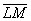,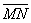, and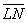.

6.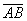and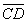bisect each other at point E. If AE = 5x - 2y + 20, BE = -4x + 12y - 24, CE = 7x - 5y + 7, and DE = 4x - 20y + 130, what are the values of x and y?

7.

Points P, Q, R, S, and T are collinear. If PT = 6PQ, QT = 15, and PQ = QR = RS, find ST.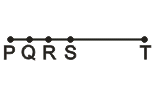8.

Points X and Y are on. If AX > BY, then which statement must be true?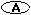AY > BX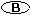AY < BY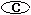AX < BX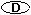XY < BY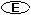AY + BX > AB

9.

Point Q is between points P and R on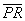. If PR = 60, PQ = 3x - 14 and RQ = 4x + 4, find the value of x.

10.

E is the midpoint of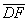. If DE = 6x - 58 and EF = 44, find the value of x.

Basics of Geometry
edHelper subscribers - Create a new printable

Number of Keys
 Select the number of different printables: 1 key 2 keys 3 keys 4 keys 5 keys

Number of Pages (for each key)
 Select the number of pages: 1 page 2 pages 3 pages 4 pages